# Chord

Point on the circle is the end point of diameter and end point of chord length of radius. What angle between chord and diameter?

Result

α =  60 °

#### Solution:Leave us a comment of example and its solution (i.e. if it is still somewhat unclear...):

Showing 0 comments:Be the first to comment!#### To solve this verbal math problem are needed these knowledge from mathematics:

See also our trigonometric triangle calculator.

## Next similar examples:

1. Two chords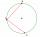There is a given circle k (center S, radius r). From point A which lies on circle k are starting two chords of length r. What angle does chords make? Draw and measure.
2. Isosceles - isoscelesIt is given a triangle ABC with sides /AB/ = 3 cm /BC/ = 10 cm, and the angle ABC = 120°. Draw all points X such that true that BCX triangle is an isosceles and triangle ABX is isosceles with the base AB.
3. CableCable consists of 8 strands, each strand consists of 12 wires with diameter d = 0.5 mm. Calculate the cross-section of the cable.
4. CircleWhat is the radius of the circle whose perimeter is 6 cm?
5. The bridge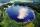Across the circle lakepasses through its center bridge over the lake. At three different locations on the lake shore are three fishermen A, B, C. Which of fishermen see the bridge under the largest angle?
6. DiagonalCan a rhombus have the same length diagonal and side?
7. QuizQ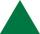An isosceles triangle has two sides of length 7 km and 39 km. How long is a third side?
8. AnglesThe outer angle of the triangle ABC at the vertex A is 114°12'. The outer angle at the vertex B is 139°18'. What size is the internal angle at the vertex C?
9. Triangle radians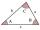The size of two internal angles of a triangle ABC are α=6/18π and β=7/18π. Calculate the size of the third angle.
10. Obtuse angleWhich obtuse angle is creating clocks at 17:00?
11. AnglesIn the triangle ABC, the ratio of angles is: a:b = 4: 5. The angle c is 36°. How big are the angles a, b?
12. Two anglesThe triangles ABC and A'B'C 'are similar. In the ABC triangle, the two angles are 25° and 65°. Explain why in the triangle A'B'C 'is the sum of two angles of 90 degrees.
13. 3-bracketMay be the largest angle in the triangle less than 20°?
14. Type of triangle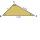How do I find the triangle type if the angle ratio is 2:3:7 ?
15. Angles in triangle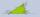Calculate the alpha angle in the triangle if beta is 61 degrees and 98 gamma degrees.
16. IronIron ore contains 57% iron. How much ore is needed to produce 20 tons of iron?
17. Center traverseIt is true that the middle traverse bisects the triangle?